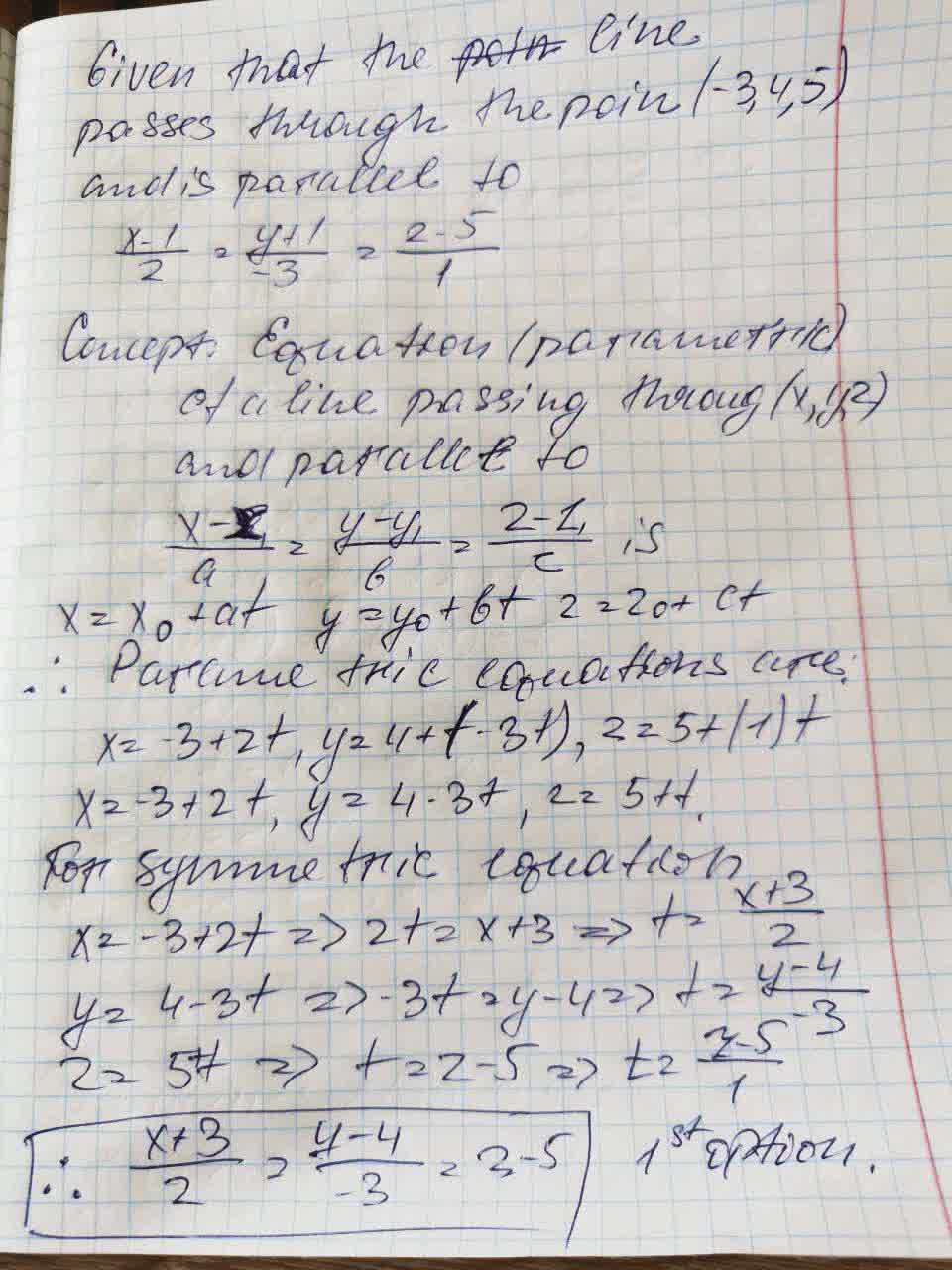Falak Kinney

2021-09-15

Find sets of parametric equations and symmetric equations of the line that passes through the given point and is parallel to the given vector or line. (For each line, write the direction numbers as integers.)
Point: (-3, 4, 5)
Parallel to: $\frac{x-1}{2}=\frac{y+1}{-3}=z-5$
(a) Parametric equations
(b) Symmetric equationsMalena